Fluid Statics

# Fluid Statics | Mechanical Engineering SSC JE (Technical)

 1 Crore+ students have signed up on EduRev. Have you?

FLUID STATICS

•  Pascal's Law 
• The intensity of pressure at any point in a stationary fluid is same in all directions.
px = py = pz
Note that pressure varies only with depth in stationary fluids, whereas if fluids is in motion pressure may vary in horizontal directions also. 
• Fluid pressure is measured in Force/ Area and it is expressed in Pascal (N/m2) or Bar.
1 Bar = 105 N/m2
1 MPa = 10 Bar 
• For static fluids, piezometric head is constant throughout the fluid.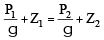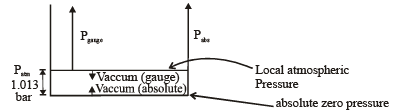• Pressure Scales
(i) Absolute Scale 
• Pressure is measured with reference to absolute zero. In this scale pressure cannot be negative. Minimum pressure is zero. 
• Abs. pressure = Pguage + local atm. pressure

(ii) Gauge Scale

• Pressure is measured with reference to atmospheric pressure. It means atmospheric pressure is taken zero.
• Guage pressure can be positive, negative or zero. 
• Gauge pressure = Absolute pressure – local atmospheric pressure 
• Vacuum pressure = local atmospheric pressure – absolute pressure 
• The atmospheric pressure varies with altitude, temperature and local conditions. It is measured by Barometer.
• At mean sea level atmospheric pressure is 1.01 × 105 Pascal or 1 Bar or 10.3 mts. of height of water or 76 cm height of mercury.
• Pressure Measuring Devices
(i) Piezometer It is simple graduated glass tube which measures pressure in gauge scale. The height up to which liquid is raised in this tube is called pressure head. It is useful for small pressure measurement and is not suitable for high pressure and negative pressure measurements.
p = gh; where 'h' is height of liquid column

(ii) Manometer It is based on liquid column balance mechanism. The manometric liquid used should have high density & low vapour pressure.
(a) Simple Manometer or U-tube Manometer
It can measure both positive and negative pressures.
(b) Differential Manometer
It measures difference in pressure between two points.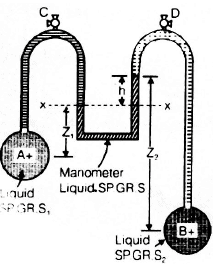(c) Micromanometer

It measures small difference of pressure. It is modified form of Simple Manometer whose one  limb is made of larger cross section area.

(d) Inclined Manometer

It measures small pressure low velocity gas flow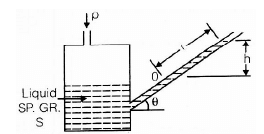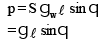S = specific gravity of liquid
gw = specific weight of water
g = specific weight of manometric liquid
(iii) Mechanical Gauges : These are used for rough measurement of high pressure. These are based on spring balance mechanism. Ex. Bourdon tube pressure gauge.
(iv) Aneroid Barometer or Mercury Barometer : It is used to measure local atmospheric pressure on absolute scale.

The document Fluid Statics | Mechanical Engineering SSC JE (Technical) is a part of the Mechanical Engineering Course Mechanical Engineering SSC JE (Technical).
All you need of Mechanical Engineering at this link: Mechanical Engineering

## Mechanical Engineering SSC JE (Technical)

6 videos|97 docs|55 tests

## Mechanical Engineering SSC JE (Technical)

6 videos|97 docs|55 tests

Track your progress, build streaks, highlight & save important lessons and more!

,

,

,

,

,

,

,

,

,

,

,

,

,

,

,

,

,

,

,

,

,

;